JEE  >  Test: Single Correct MCQs: Straight Lines and Pair of Straight Lines | JEE Advanced

# Test: Single Correct MCQs: Straight Lines and Pair of Straight Lines | JEE Advanced

Test Description

## 23 Questions MCQ Test Mathematics For JEE | Test: Single Correct MCQs: Straight Lines and Pair of Straight Lines | JEE Advanced

Test: Single Correct MCQs: Straight Lines and Pair of Straight Lines | JEE Advanced for JEE 2023 is part of Mathematics For JEE preparation. The Test: Single Correct MCQs: Straight Lines and Pair of Straight Lines | JEE Advanced questions and answers have been prepared according to the JEE exam syllabus.The Test: Single Correct MCQs: Straight Lines and Pair of Straight Lines | JEE Advanced MCQs are made for JEE 2023 Exam. Find important definitions, questions, notes, meanings, examples, exercises, MCQs and online tests for Test: Single Correct MCQs: Straight Lines and Pair of Straight Lines | JEE Advanced below.
Solutions of Test: Single Correct MCQs: Straight Lines and Pair of Straight Lines | JEE Advanced questions in English are available as part of our Mathematics For JEE for JEE & Test: Single Correct MCQs: Straight Lines and Pair of Straight Lines | JEE Advanced solutions in Hindi for Mathematics For JEE course. Download more important topics, notes, lectures and mock test series for JEE Exam by signing up for free. Attempt Test: Single Correct MCQs: Straight Lines and Pair of Straight Lines | JEE Advanced | 23 questions in 50 minutes | Mock test for JEE preparation | Free important questions MCQ to study Mathematics For JEE for JEE Exam | Download free PDF with solutions
 1 Crore+ students have signed up on EduRev. Have you?
Test: Single Correct MCQs: Straight Lines and Pair of Straight Lines | JEE Advanced - Question 1

### The points (–a, – b), (0, 0), (a, b) and (a2, ab) are : (1979)

Detailed Solution for Test: Single Correct MCQs: Straight Lines and Pair of Straight Lines | JEE Advanced - Question 1

The given points are A (– a, – b), B (0, 0), C (a, b) and D (a2, ab).
Slope of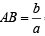Slope of BC = slope of BD

∴ A, B, C, D are collinear.

Test: Single Correct MCQs: Straight Lines and Pair of Straight Lines | JEE Advanced - Question 2

### Th e poin t (4, 1) under goes th e following t hr ee transformations successively. (1980) (i) Reflection about the line y = x. (ii) Translation through a distance 2 units along the positive direction of x-axis. (iii) Rotation through an angle p/4 about the origin in the counter clockwise direction. Then the final position of the point is given by the coordinates.

Detailed Solution for Test: Single Correct MCQs: Straight Lines and Pair of Straight Lines | JEE Advanced - Question 2

Reflection about the line y = x, changes the point (4, 1) to (1, 4).
On translation of (1, 4) through a distance of 2 units along +ve direction of x-axis the point becomes (1 + 2, 4), i.e., (3, 4).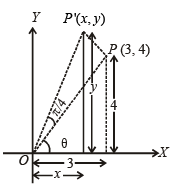On rotation about origin through an angle p/4 the point P takes the position P' such that OP = OP'

Also OP = 5 = OP' and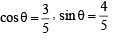Now, x = OP'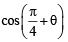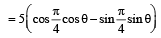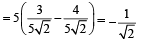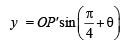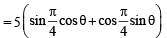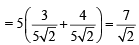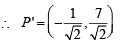Test: Single Correct MCQs: Straight Lines and Pair of Straight Lines | JEE Advanced - Question 3

### The straight lines x + y = 0, 3x + y – 4 = 0, x + 3y – 4 = 0 form a triangle which is (1983 - 1 Mark)

Detailed Solution for Test: Single Correct MCQs: Straight Lines and Pair of Straight Lines | JEE Advanced - Question 3

Solving the given equations of lines pairwise, we get the vertices of Δ as A (– 2, 2) B (2, – 2), C (1, 1)

Then AB  =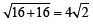BC =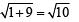CA =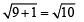∴   Δ is isosceles.

Test: Single Correct MCQs: Straight Lines and Pair of Straight Lines | JEE Advanced - Question 4

If P = (1, 0), Q = (–1, 0) and R = (2, 0) are three given points, then locus of the point S satisfying the relation SQ2 + SR2 = 2SP2,  is (1988 - 2 Marks)

Detailed Solution for Test: Single Correct MCQs: Straight Lines and Pair of Straight Lines | JEE Advanced - Question 4

We have P = (1, 0), Q = (– 1, 0), R = (2, 0)
Let   S = (x, y) ATQ   SQ2 + SR2 = 2SP2
⇒ (x + 1)2 + y2 + (x – 2)2 + y2 = 2[(x – 1)2 + y2]
⇒ 2x2 + 2y2 – 2x + 5 = 2x2 + 2y– 4x + 2
⇒ 2x + 3 = 0 ⇒ x = – 3/2
Which is a straight line parallel to y-axis.

Test: Single Correct MCQs: Straight Lines and Pair of Straight Lines | JEE Advanced - Question 5

Line L has intercepts a and b on the coordinate axes. When the axes are rotated through a given angle, keeping the origin fixed, the same line L has intercepts p and q, then (1990 -  2 Marks)

Detailed Solution for Test: Single Correct MCQs: Straight Lines and Pair of Straight Lines | JEE Advanced - Question 5

As L has intercepts a and b on axes, equation of L is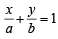.....… (1)
Let x and y axes be rotated through an angle q in anticlockwise direction.
In new system intercepts are p and q, therefore equation of L becomes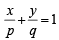.....… (2)
KEY CONCEPT :  As the origin is fixed in rotation, the distance of line from origin in both the cases should be same.

∴ We get  d =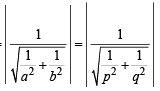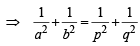∴ (b) is the correct answer.

Test: Single Correct MCQs: Straight Lines and Pair of Straight Lines | JEE Advanced - Question 6

If the sum of the distances of a point from two perpendicular lines in a plane is 1, then its locus is (1992 -  2 Marks)

Detailed Solution for Test: Single Correct MCQs: Straight Lines and Pair of Straight Lines | JEE Advanced - Question 6

Let the two perpendicular lines be the co-ordinate axes.
Let (x, y) be the point sum of whose distances from two axes is 1 then we must have | x | + | y | = 1  or ± x ± y = 1
These are the four lines x + y = 1, x – y = 1, – x + y = 1, – x – y = 1

Any two adjacent sides are perpendicular to each other.
Also each line is equidistant from origin. Therefore figure formed is a square.

Test: Single Correct MCQs: Straight Lines and Pair of Straight Lines | JEE Advanced - Question 7

The locus of a variable point whose distance from (–2, 0) is 2/3 times its distance from the line x = -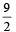is (1994)

Detailed Solution for Test: Single Correct MCQs: Straight Lines and Pair of Straight Lines | JEE Advanced - Question 7

If variable point is P and S (– 2, 0) then  PS =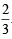PM where PM is the perpendicular distance of point P from given line x = – 9/2

∴ By definition P describes an ellipse.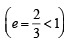Test: Single Correct MCQs: Straight Lines and Pair of Straight Lines | JEE Advanced - Question 8

The equation s to a pair of opposite sides of parallelogram are x2 – 5x + 6 = 0 and y2 – 6y + 5 = 0, the equations to its diagonals are (1994)

Detailed Solution for Test: Single Correct MCQs: Straight Lines and Pair of Straight Lines | JEE Advanced - Question 8

The sides of parallelogram are x = 2, x = 3, y = 1, y = 5.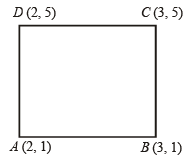∴ Diagonal AC is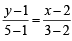or y = 4x – 7

Equation diagonal BD is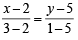or 4x + y = 13

Test: Single Correct MCQs: Straight Lines and Pair of Straight Lines | JEE Advanced - Question 9

The orthocentre of the triangle formed by the lines xy = 0 and x + y = 1 is (1995S)

Detailed Solution for Test: Single Correct MCQs: Straight Lines and Pair of Straight Lines | JEE Advanced - Question 9

The lines by which Δ is formed are x = 0, y = 0 and x + y = 1.
Clearly, it is right Δ and we know that in a right Δ orthocentre coincides with the vertex at which right ∠ is formed.
∴ Orthocentre is (0, 0).

Test: Single Correct MCQs: Straight Lines and Pair of Straight Lines | JEE Advanced - Question 10

Let PQR be a right angled isosceles triangle, right angled at P (2, 1). If the equation of the line QR is 2x + y = 3, then the equation representing the pair of lines PQ and PR is (1999 - 2 Marks)

Detailed Solution for Test: Single Correct MCQs: Straight Lines and Pair of Straight Lines | JEE Advanced - Question 10

Let m be the slope of PQ then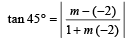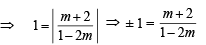⇒ m + 2 = 1 – 2m or – 1 + 2m = m + 2
⇒ m = – 1/3 or m = 3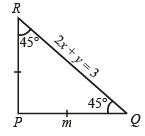As PR also makes ∠ 45° with RQ.
∴ The above two values of m are for PQ and PR.

∴ Equation of PQ,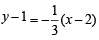⇒ 3y – 3 = – x + 2 ⇒ x + 3y – 5 = 0

and equation of PR is ⇒ 3x – y – 5 = 0
∴ Combined equation of PQ and PR is (x – 3y – 5) (3x – y – 5) = 0
⇒ 3x2 – 3y+ 8xy – 20x – 10y + 25 = 0

Test: Single Correct MCQs: Straight Lines and Pair of Straight Lines | JEE Advanced - Question 11

If x1, x2, x3 as well as y1, y2, y3,  are in G.P. with the same common ratio, then the points (x1, y1), (x2, y2) and (x3, y3). (1999 - 2 Marks)

Detailed Solution for Test: Single Correct MCQs: Straight Lines and Pair of Straight Lines | JEE Advanced - Question 11

x2 = x1r, x3 = x1r2 and so is y= y1r, y3 = y1r2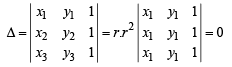Hence the points lie on a line, i.e., they are collinear.

Test: Single Correct MCQs: Straight Lines and Pair of Straight Lines | JEE Advanced - Question 12

Let PS be the median of the triangle with vertices P(2, 2), Q(6, –1) and R(7, 3). The equation of the line passing through (1,–1) and parallel to PS is (2000S)

Detailed Solution for Test: Single Correct MCQs: Straight Lines and Pair of Straight Lines | JEE Advanced - Question 12

S is the midpoint of Q and R

Therefore,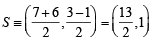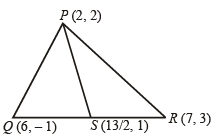Now slope of  PS = m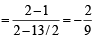Now equation of the line passing through (1, – 1) and parallel to PS is

y + 1 =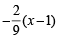or 2x + 9y + 7 = 0

Test: Single Correct MCQs: Straight Lines and Pair of Straight Lines | JEE Advanced - Question 13

The incentre of the triangle with vertices (1, √3 ), (0, 0) and (2, 0) is                       (2000S)

Detailed Solution for Test: Single Correct MCQs: Straight Lines and Pair of Straight Lines | JEE Advanced - Question 13

Here AB = BC = CA = 2. So, it is an equilaterial triangle and the incentre coincides with centroid.
Therefore,

Incentre = Centroid =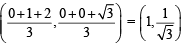Test: Single Correct MCQs: Straight Lines and Pair of Straight Lines | JEE Advanced - Question 14

The number of integer values of m, for which the x-coordinate of the point of intersection of the lines 3x + 4y = 9 and y = mx + 1 is also an integer, is (2001S)

Detailed Solution for Test: Single Correct MCQs: Straight Lines and Pair of Straight Lines | JEE Advanced - Question 14

Intersection of 3x + 4y = 9 and y = mx + 1.
For x co-ordinate 3x + 4 (mx + 1) = 9 ⇒ (3 + 4m) x = 5For x to be an integer 3 + 4m should be a divisor of 5 i.e., 1, – 1, 5 or – 5. 3 + 4m = 1
⇒ m = – 1/2 (not integer) 3 + 4m = – 1 ⇒ m = – 1 (integer) 3 + 4m =  5
⇒ m = 1/2 (not an integer) 3 + 4m = – 5 ⇒ m = – 2 (integer)
∴ There are 2 integral values of m.
∴ (a) is the correct alternative.

Test: Single Correct MCQs: Straight Lines and Pair of Straight Lines | JEE Advanced - Question 15

Area of the parallelogram formed by the lines y = mx, y = mx + 1, y = nx and y = nx + 1 equals (2001S)

Detailed Solution for Test: Single Correct MCQs: Straight Lines and Pair of Straight Lines | JEE Advanced - Question 15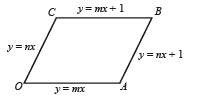The vertices,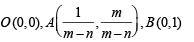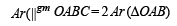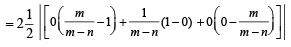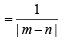Test: Single Correct MCQs: Straight Lines and Pair of Straight Lines | JEE Advanced - Question 16

Let P = (-1, 0), Q = (0, 0) and R = (3, 33 ) be three points.
Then the equation of the bisector of the angle PQR is                (2002S)

Detailed Solution for Test: Single Correct MCQs: Straight Lines and Pair of Straight Lines | JEE Advanced - Question 16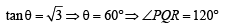⇒ bisector will have slope tan 120°
⇒ equation of bisector is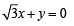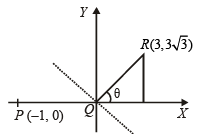Test: Single Correct MCQs: Straight Lines and Pair of Straight Lines | JEE Advanced - Question 17

Let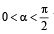be fixed angle. If     P = (cos q, sin q) and Q = (cos(a - q), sin(a - q)) , then Q is obtained from P by (2002S)

Detailed Solution for Test: Single Correct MCQs: Straight Lines and Pair of Straight Lines | JEE Advanced - Question 17

Clearly OP = OQ = 1 and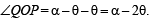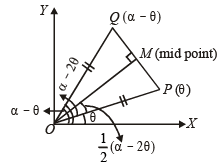The bisector of ∠QOP will be a perpendicular to PQ and also bisect it. Hence Q is reflection of P in the line OM which makes an angle ∠MOP + ∠POX with x- axis,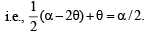So that slope of OM is tan a/2.

Test: Single Correct MCQs: Straight Lines and Pair of Straight Lines | JEE Advanced - Question 18

A straight line through the origin O meets the parallel lines 4x + 2y = 9 and 2x + y + 6 = 0 at points P and Q respectively.Then the point O divides the segemnt PQ in the ratio (2002S)

Detailed Solution for Test: Single Correct MCQs: Straight Lines and Pair of Straight Lines | JEE Advanced - Question 18

The given lines are 2x + y = 9/2 .....… (1)
and 2x + y = – 6 .....… (2)
Signs of constants on R.H.S. show that two lines lie on opp. sides of origin. Let any line through origin meets these lines in P and Q respectively then req. ratio is OP : OQ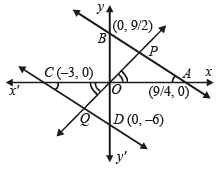Now in DOPA and DOQC, Ð POA = Ð QOC (ver. opp. Ð' s) Ð PAO = Ð OCQ (alt. int. Ð' s) ∠
∴DOPA ~ DOQC (by AA similarly)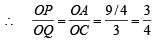∴ Req. ratio is 3 : 4.

Test: Single Correct MCQs: Straight Lines and Pair of Straight Lines | JEE Advanced - Question 19

The number of intergral points (integral point means both the coordinates should be integer) exactly in the interior of the triangle with vertices (0,0), (0,21) and (21,0), is (2003S)

Detailed Solution for Test: Single Correct MCQs: Straight Lines and Pair of Straight Lines | JEE Advanced - Question 19

Total no. of points within the square OABC = 20 × 20 = 400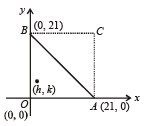Points on line AB = 20 ((1, 1), (2, 2), ....… (20, 20))
∴ Points within DOBC and DABC = 400 – 20 = 380
By symmetry points within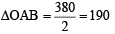Test: Single Correct MCQs: Straight Lines and Pair of Straight Lines | JEE Advanced - Question 20

Orthocentre of triangle with vertices (0, 0), (3, 4) and (4, 0) is (2003S)

Detailed Solution for Test: Single Correct MCQs: Straight Lines and Pair of Straight Lines | JEE Advanced - Question 20

We know that or thocen tre is the meeting point of altitudes of a Δ.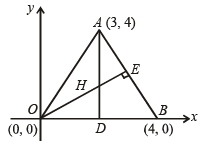⇒ line parallel to y-axis through (3, 4)
⇒ x = 3 ......... (1)
Similarly eqn of OE ⊥ AB is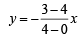⇒ y = x/4 ......... (2)

Solving (1) and (2), we get orthocentre as (3, 3/4)

Test: Single Correct MCQs: Straight Lines and Pair of Straight Lines | JEE Advanced - Question 21

Area of the triangle formed by th e lin e x + y = 3 an d an gle bisectors of the pair of straight lines x2 – y2 + 2y = 1 is (2004S)

Detailed Solution for Test: Single Correct MCQs: Straight Lines and Pair of Straight Lines | JEE Advanced - Question 21

x– y2 + 2y = 1  ⇒ x = ± (y – 1)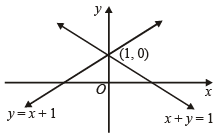Bisectors of above lines are x = 0 and y = 1.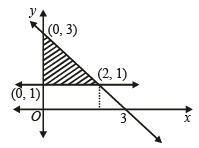So area between x = 0, y = 1 and x + y = 3 is shaded region shown in figure.

Area  =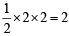sq. units.

Test: Single Correct MCQs: Straight Lines and Pair of Straight Lines | JEE Advanced - Question 22

Let O(0, 0), P(3, 4), Q(6, 0) be the vertices of the triangles OPQ. The point R inside the triangle OPQ is such that the triangles OPR, PQR, OQR are of equal area. The coordinates of R are (2007 -3 marks)

Detailed Solution for Test: Single Correct MCQs: Straight Lines and Pair of Straight Lines | JEE Advanced - Question 22

∵ Ar (ΔOPR) = Ar (ΔPQR) = Ar (ΔOQR)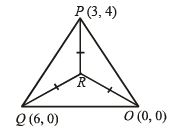∴ By simply geometry R should be the centroid of ΔPQO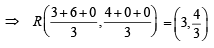Test: Single Correct MCQs: Straight Lines and Pair of Straight Lines | JEE Advanced - Question 23

A straight line L through the point (3, –2) is inclined at an angle 60° to the line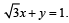If L also intersects the x-axis, then the equation of L is     (2011)

Detailed Solution for Test: Single Correct MCQs: Straight Lines and Pair of Straight Lines | JEE Advanced - Question 23

Let the slope of line L be m.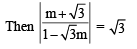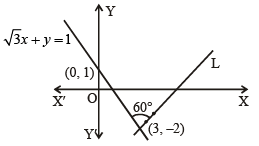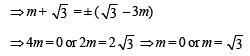∵ L intersects x-axis,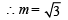∴ Equation of L is y + 2 =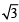(x – 3)

or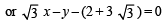## Mathematics For JEE

130 videos|359 docs|306 tests
 Use Code STAYHOME200 and get INR 200 additional OFF Use Coupon Code
Information about Test: Single Correct MCQs: Straight Lines and Pair of Straight Lines | JEE Advanced Page
In this test you can find the Exam questions for Test: Single Correct MCQs: Straight Lines and Pair of Straight Lines | JEE Advanced solved & explained in the simplest way possible. Besides giving Questions and answers for Test: Single Correct MCQs: Straight Lines and Pair of Straight Lines | JEE Advanced, EduRev gives you an ample number of Online tests for practice

## Mathematics For JEE

130 videos|359 docs|306 tests